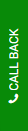# Negative Interest Rate as Method of Monetary Policy To Boost Bank Economy Assessment AnswerThe negative interest rate has been proposed as a unique method of monetary policy to boost
banks’ lending to stimulate the economy.

Introduction
A negative interest rate policy (NIRP) is an unusual monetary policy, meaning that commercial banks and other deposit taking financial institutions, must pay interest to deposit their excess reserves at the Central Bank. The 2008 GFC forced many central banks to cut interest rates close to zero. With an ongoing economic downturn European Central Bank reduced the key lending rate below zero in 2014. Japan followed the same policy after that. We are now in the era of negative interest rates, and it is the subject of debate as to whether this monetary policy has distorted financial markets, increased financial risk, and may discourage the public to park their money in the deposit taking financial institutions.
Question 1: Define the real and nominal interest rates, then show their relationship in a formula. What is the difference between the real and nominal NEGATIVE interest rates? What is the
difference between these two if a central bank wants to use them as a tool for monetary policy? Use sub-headings to explain each point.

Requirements

Write an 8-page assignment (excluding references) with sub-headings for each question. Each question can be around one and half  page though you’ll answer some in much less space than others. You
can go above or below the suggestion. However, the total page limit must be adhered to

Extra evidence such as tables and graphs are highly desirable. They must be placed in the text.

The figures or tables should be placed directly above or wrapped in the paragraph that they are discussed in. Do not put figures in the appendix; they will not be marked.

• The main body of your assignment should not exceed the page limit, typed with 12-point Arial font, 1.5 spaced with default margins. This page is formatted in this way. Exceeding the page count will incur a one-mark penalty for each half page. The bibliography or reference list is not included in the word count, but any appendices will be. Please refrain from including an appendix since you should not require it, all tables and graphs should be included in-text next to where they are discussed.

• A good assignment shows research and clarity of argument.

• Assignments that are not proof-read will be marked down. Grammar and sentence structure is important.

• Answer the questions in the order that they are asked. Be sure to include subheadings that contain the question number.

• There's no need for an introduction or conclusion. This assignment is a collection of short answer questions

• There's no need to write the whole question in your assignment if you're struggling to fit everything into the page limit. You may write the question number as a subtitle to show where
each question begins. For example:

# QUESTION 1

Real and Nominal Interest rate

Real interest rate is defined as interest rate which has adjusted in order to remove effects of inflation for reflecting real cost of funding to borrowers as well as real yielding to lender or investor (Assenmacher and Krogstrup, 2018).  This interest rate focuses on reflecting rate of time preference for existing products over future time period of goods. Main benefits is rise in interest rate leads to pay lower interest loans whereas drawback is high interest rate leads to drive up monthly mortgage amount beyond limit.Figure 1: Real Interest Rate 2020

(Source: Real Interest rate of Government Bonds, 2020)

On other hand, nominal interest rate is regarded as interest rate which is before taking of inflation into consideration (Forbes, Reinhardt and Wieladek, 2017). This is regarded as stated or advertised interest rate on provided loan without taking account of compounding as well as fees of interest. For example, if person has borrowed \$100 at rate of 6% interest then they are liable to pay \$6 on given amount which does not take inflation into consideration. Main drawbacks of such rate are that it does not consider adjusting inflation rate.

Relationship between both in formula

Nominal Interest rate= Real Interest + Expected Inflation

Real Interest Rate= Nominal Interest Rare- Expected Inflation

Difference between real and nominal negative interest rates

Real negative interest rate means inflation rate is higher than nominal interest rate. Whenever there is negative value of interest then it leads to rise in inflation rate which is greater than nominal rate (Hanisch, 2017). For example, if rate of federal rate is 2% as well as inflation is 10% then borrower profit will be 7.27% of each dollar in borrowed amount every year.Figure 2: Real Interest Rate, 2020

(Source: Gold in the Negative Real Interest Rates Environment, 2020)

On other side, nominal negative interest rates cannot be negative due to bank charging negative same interest rate as they will pay money to borrowers (Khan, Ahmad and Gee, 2016). These are set with value of negative due to theoretical lower bound which is zero percent. It is not possible to have negative nominal interest rate.

Describing both

If central bank wants to use as tool for monetary policy then they real interest rate can be negative whereas nominal rate is not negative as they have to pay money to borrower which is negative point for it. This is not possible to make value of nominal rate to be zero where as interest rate can be zero and leads to measure value of rate. Therefore, they will use real interest rate for monetary policy (Chen, Ren and Zha, 2018)

From side of lowering borrowing costs, negative rate assist in weakening currency of country through making it less attractive investment than various types of currencies. This provides country helps in exporting competitive advantage as well as boosting inflation through pushing more cost of imports for business. It will lead to provide advantage to business in positive manner and leads towards success and growth of country in positive manner. Thus, country prefers to have real negative interest rate for boosting economy of country and used as monetary policy for positive benefits.